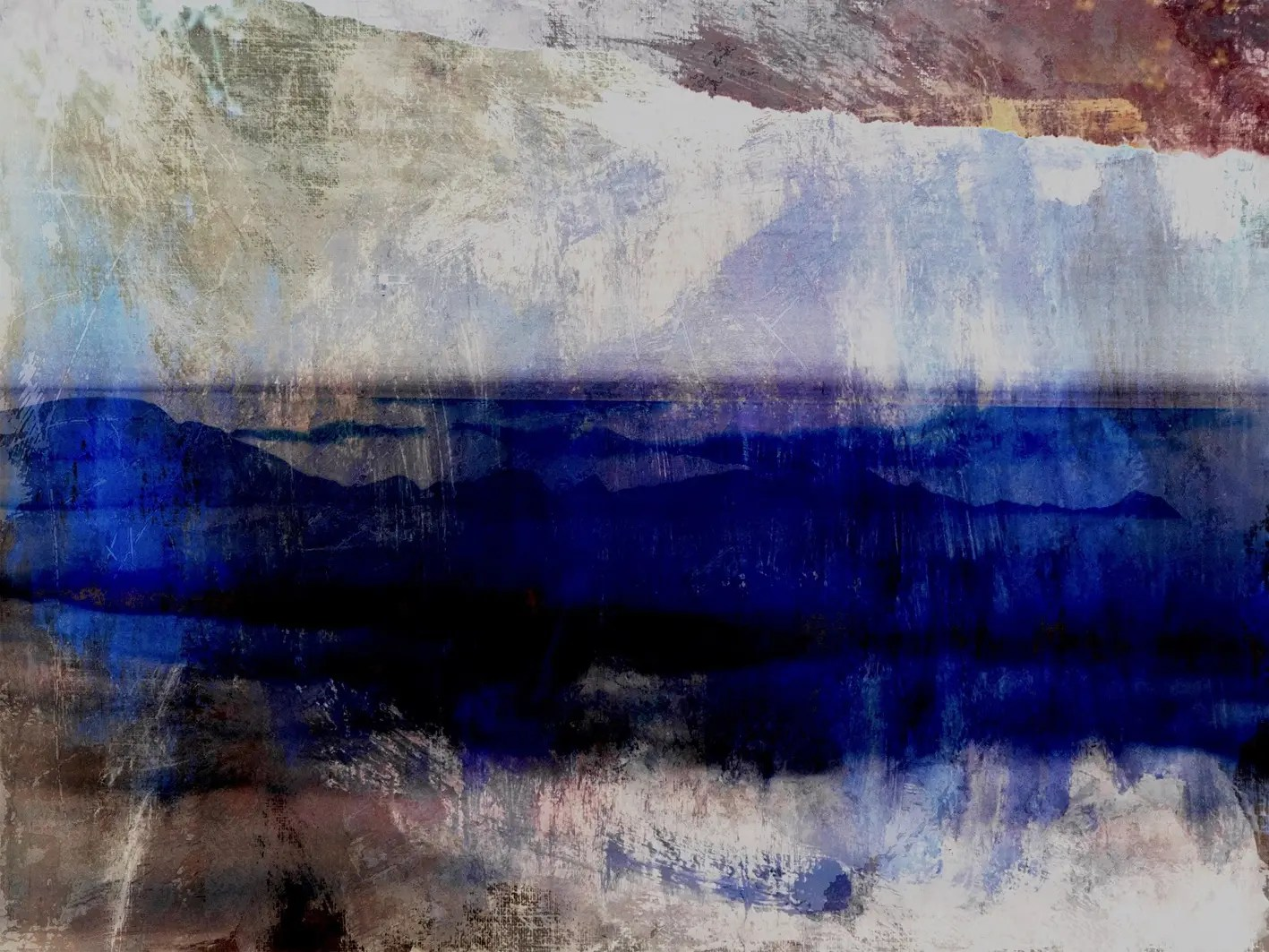• ## SELECT A CATEGORY##### Currency Conversion from ZAR to:
[convert number=25000.00 from="zar" to="usd"] USD | [convert number=25000.00 from="zar" to="gbp"] GBP | [convert number=25000.00 from="zar" to="eur"] EUR
Artist: [popup_anything id="428902"]   Medium: Oil on Canvas   Size: 90cm by 120cm

R 25,000.00##### Currency Conversion from ZAR to:
[convert number=25000.00 from="zar" to="usd"] USD | [convert number=25000.00 from="zar" to="gbp"] GBP | [convert number=25000.00 from="zar" to="eur"] EUR
Artist: [popup_anything id="428906"]   Medium: Oil on Canvas   Size: 90cm by 120cm
R 25,000.00##### Currency Conversion from ZAR to:
[convert number=9200.00 from="zar" to="usd"] USD | [convert number=9200.00 from="zar" to="gbp"] GBP | [convert number=9200.00 from="zar" to="eur"] EUR
Artist: [popup_anything id="428896"]   Medium: Archival Print on Canvas   Size: 120cm by 90cm   Edition: 500
R 9,200.00##### Currency Conversion from ZAR to:

[convert number=36000.00 from="zar" to="usd"] USD | [convert number=36000.00 from="zar" to="gbp"] GBP | [convert number=36000.00 from="zar" to="eur"] EUR |

[convert number=36000.00 from="zar" to="zar"] ZAR
Artist: [popup_anything id="336020"] Medium: Oil on Canvas Size: 127cm by 101,5cm
SOLD##### Currency Conversion from ZAR to:
[convert number=24000.00 from="zar" to="usd"] USD | [convert number=24000.00 from="zar" to="gbp"] GBP | [convert number=24000.00 from="zar" to="eur"] EUR | [convert number=24000.00 from="zar" to="zar"] ZAR   Artist: [popup_anything id="336020"] Medium: Oil on Canvas Size: 122.5cm by 91,5cm SOLD##### Currency Conversion from ZAR to:
[convert number=37000.00 from="zar" to="usd"] USD | [convert number=37000.00 from="zar" to="gbp"] GBP | [convert number=37000.00 from="zar" to="eur"] EUR
Artist: [popup_anything id="336020"] Medium: Acrylic on Canvas Size: 196,5cm by 148cm SOLD##### Currency Conversion from ZAR to:
[convert number=12500.00 from="zar" to="usd"] USD | [convert number=12500.00 from="zar" to="gbp"] GBP | [convert number=12500.00 from="zar" to="eur"] EUR
Artist: [popup_anything id="336020"] Medium: Digital Collage on Perspex Size: 80cm by 110cm Edition: 100
R 12,500.00##### Currency Conversion from ZAR to:
[convert number=19500.00 from="zar" to="usd"] USD | [convert number=19500.00 from="zar" to="gbp"] GBP | [convert number=19500.00 from="zar" to="eur"] EUR
Artist: [popup_anything id="336020"] Medium: Oil on Canvas Size: 80cm by 90cm
R 19,500.00##### Currency Conversion from ZAR to:
[convert number=21000.00 from="zar" to="usd"] USD | [convert number=21000.00 from="zar" to="gbp"] GBP | [convert number=21000.00 from="zar" to="eur"] EUR
Artist: [popup_anything id="336020"] Medium: Oil on Canvas Size: 122cm by 80cm
R 21,000.00##### Currency Conversion from ZAR to:
[convert number=22700.00 from="zar" to="usd"] USD | [convert number=22700.00 from="zar" to="gbp"] GBP | [convert number=22700.00 from="zar" to="eur"] EUR
Artist: [popup_anything id="336020"] Medium: Oil on Canvas Size: 100cm by 100cm
R 22,700.00##### Currency Conversion from ZAR to:
[convert number=24000.00 from="zar" to="usd"] USD | [convert number=24000.00 from="zar" to="gbp"] GBP | [convert number=24000.00 from="zar" to="eur"] EUR
Artist: [popup_anything id="336020"] Medium: Oil on Canvas Size: 92cm by 153cm
R 24,000.00##### Currency Conversion from ZAR to:
[convert number=24000.00 from="zar" to="usd"] USD | [convert number=24000.00 from="zar" to="gbp"] GBP | [convert number=24000.00 from="zar" to="eur"] EUR
Artist: [popup_anything id="336020"] Medium: Oil on Canvas Size: 127cm by 102cm
R 24,000.00